# Integral Calculus   Share

## What is Integral Calculus

In Mathematics, Integral Calculus is one of the very important topics to understand, the difficulty of question from this topic is medium and will be easy to solve if you are through with the concept. Every year you will get at max 1 - 3 questions in JEE Main and other exams, directly (as chapter weight in jee main is only 9%) but indirectly, the application of this chapter will be involved in physics and area finding problems. This chapter will be helpful in mechanics to solve the problem which involves integration. It will be a new chapter for student and you will find it difficult to learn initially but with time once you understand the basic concept and do the proper amount of practice you will find it handy. This chapter is highly calculative, so you need to do proper practice, you should solve an ample amount of questions and make sure you are good with calculations. Overall this chapter will be larger than other chapters as it has three topics combined.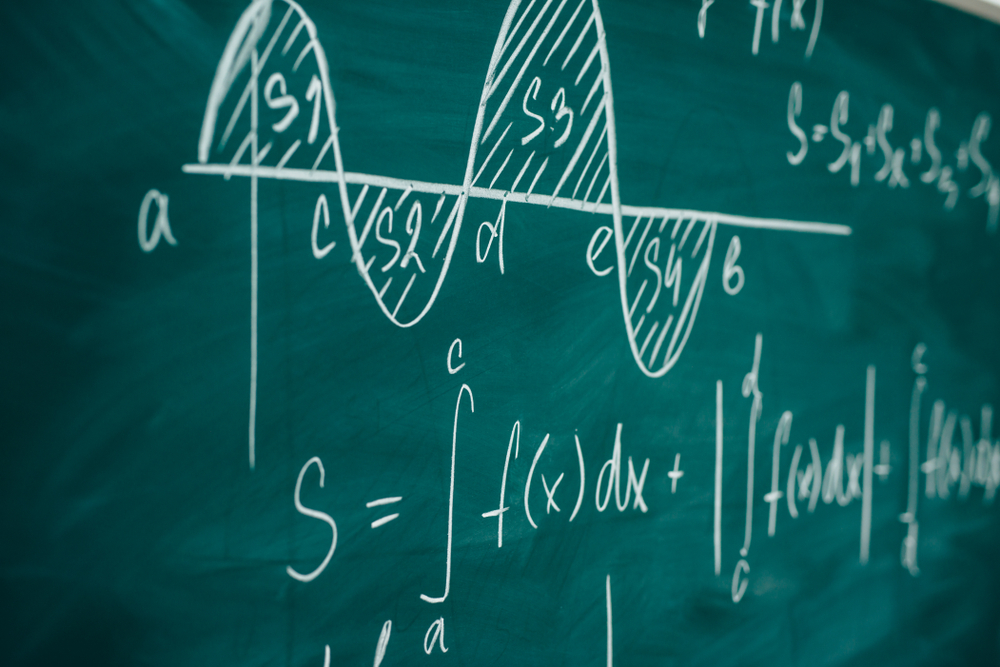## Why Integral Calculus:

Integral Calculus is important due to various real-life cases and the handy tool it provides for various real-life application. It is used in finance to price bonds and options, credit card companies use integral calculus to set the due on credit cards. In chemistry, the rate of reaction is determined by using the integral calculus. The area finding the application of integral calculus is used by architect-engineer to determine the need for material for the construction. Graphic design artists use integral calculus to determine how different three-dimensional model will behave when subjected to rapidly changing conditions.

If you understand this chapter well you will realize how good tools this chapter provide to you. You will love to solve questions based on this chapter.

## After studying Integral Calculus:

1. After studying this chapter you will find new tools of mathematics which you will be exploring, and maybe you will enjoy using it.
2. You will feel comfortable with the calculative problem of mechanics in physics which involves integration.
3. This chapter will give you a different approach of thinking to solve a problem in physics and in general in academic research which will make you more equipped to deal with the problem and hence confident.
4. And obviously, the chapter itself will help you to score some marks in the exam as it gets about 9% weight in jee main.
Prepare Online for JEE Main/NEET

Crack JEE 2021 with JEE/NEET Online Preparation Program

## Notes on Integral Calculus:

Important topics :

1. Introduction, integration as the inverse function of differentiation

2. Indefinite integral and properties of indefinite integral

3. Comparison between definite and indefinite integration

4. Methods of integration, Integration by substitution, integration using trigonometric identities, integration by partial fractions, integration by parts, etc

5. Integrals of some particular function

6. Integral of some special types

7. Definite integral and its properties

8. The fundamental theorem of calculus

9. Evaluations of definite integral by substitution

## Overview of the Integral Calculus:

Introduction: Differential Calculus is centered on the concept of the derivative. The original motivation for the derivative was the problem of defining tangent lines to the graphs of functions and calculating the slope of such lines. Integral Calculus is motivated by the problem of defining and calculating the area of the region bounded by the graph of the functions.

a) The problem of finding a function whenever its derivative is given,

b) The problem of finding the area bounded by the graph of a function under certain conditions.

These two problems lead to the two forms of the integrals, e.g., indefinite and definite integrals, which together constitute the Integral Calculus.

There is a connection, known as the Fundamental Theorem of Calculus, between indefinite integral and definite integral which makes the definite integral as a practical tool for science and engineering

Definite integral:  If a function f isdifferentiableinanintervalI, i.e., its derivative f ′ exists at each point of I, then a natural question arises that given f ′ at each point of I, can we determine the function? The functions that could possibly have given

a function as a derivative are called antiderivatives (or primitive) of the function. Further, the formula that gives all these antiderivatives is called the indefinite integral of the function and such process of finding antiderivatives is called integration.

## Methods of integration

Integration using substitution: this method uses the concept of change of variables in which we substitute some other function for the existing function to make the integration easy (by putting the other function as a substitution of the independent variable),  given integrations is transformed to some easy integrations using and then integrated.

For example let then we substitute x  = g(t)

Differentiating x = g(t) both sides we get dx = g′(t) dt

Integration by parts: this method is quite useful when we integrate product of functions If u and v are any two differentiable functions of a single variable x (say). Then, by the product rule of differentiation, we have

d (uv) = u dv + v du

Integrating both sides, we get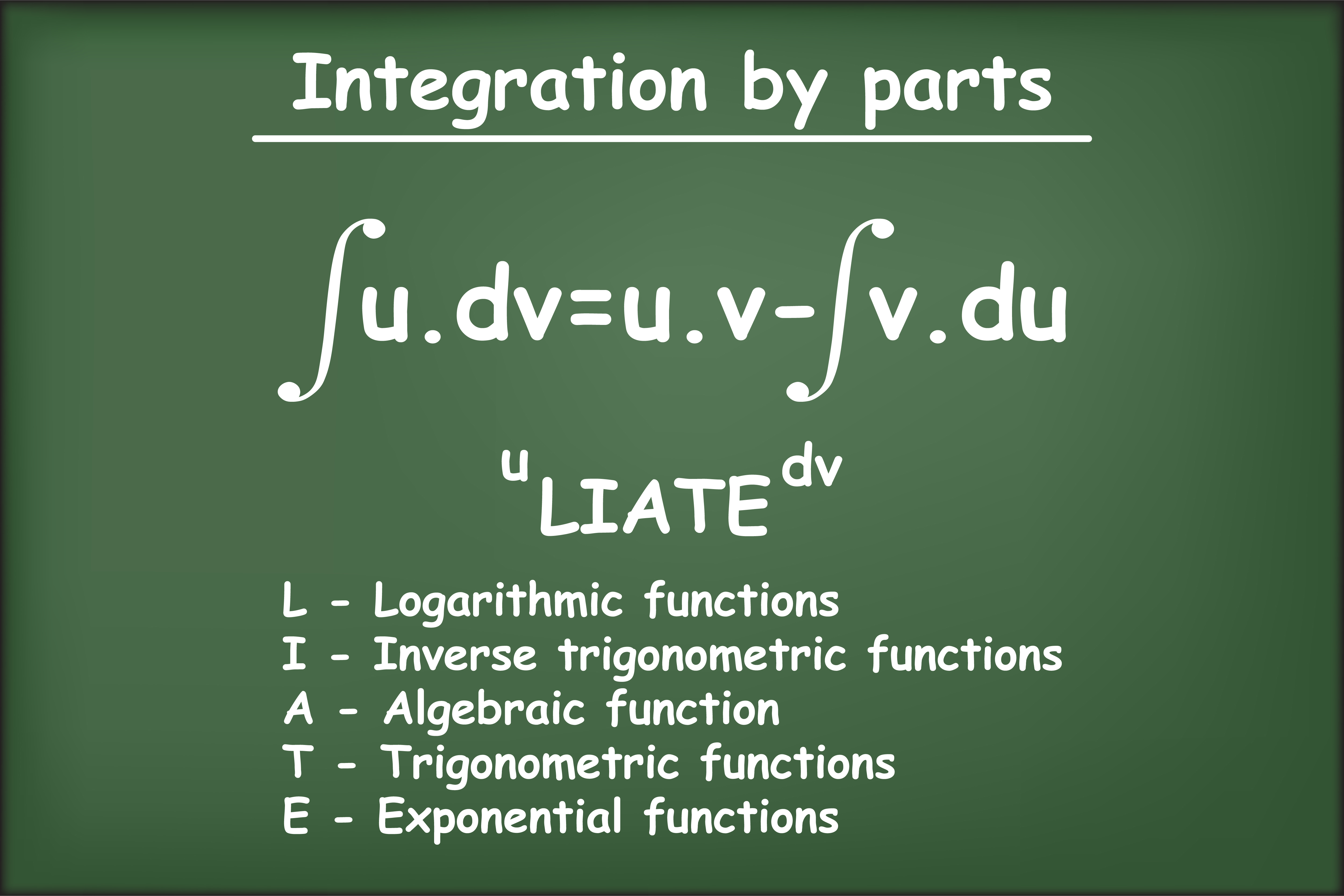or

If we take f as the first function and g as the second function, then this formula may be stated as follows:

“The integral of the product of two functions = (first function) × (integral of the second function) – Integral of [(differential coefficient of the first function)× (integral of the second function)]”

Integral of some particular functions: integral of the type

We have

Thus,

Definite integrals: The definite integral has a unique value. A definite integral is denoted by  , where a is called the lower limit of the integral and b is called the upper limit of the integral. The definite integral is introduced either as the limit of a sum or if it has an antiderivative F in the interval [a, b], then its value is the difference between the values of F at the end points, i.e., F(b) – F(a)

Fundamental theorem of calculus:

First fundamental theorem of calculus: Let f be a continuous function on the closed interval [a, b] and let A (x) be the area function. Then A′(x) = f (x), for all x ∈ [a, b].

The second fundamental theorem of calculus: Let f be a continuous function defined on the closed interval [a, b] and F be an anti derivative of f. Then

.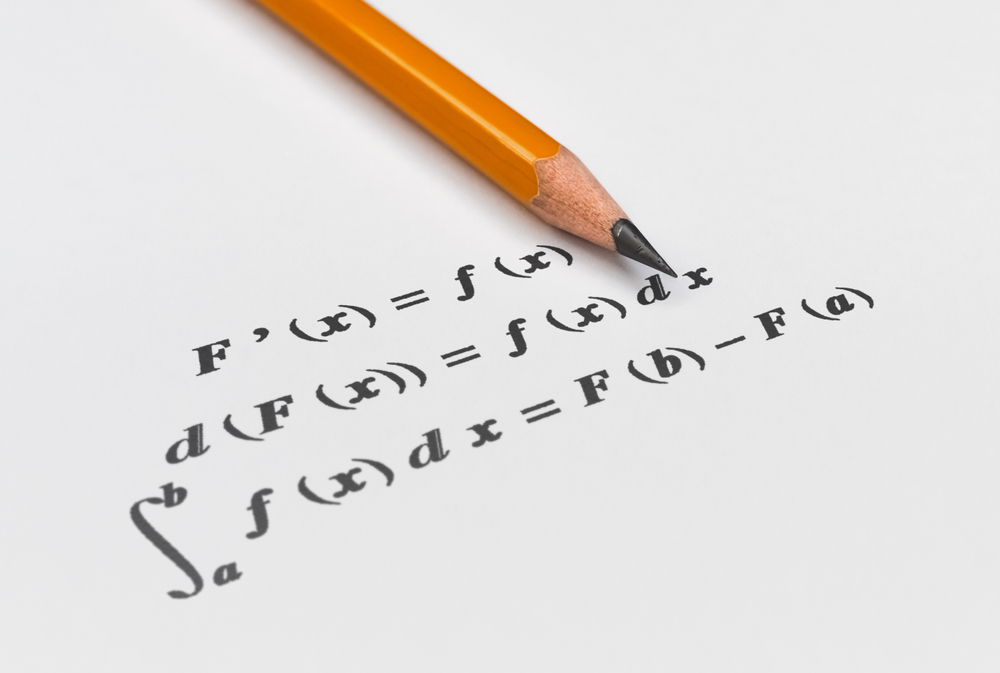Evaluation of definite integral by substitution: To evaluate   , by substitution, the steps could be as follows:

1. Consider the integral without limits and substitute, y = f (x) or x = g(y) to reduce the given integral to a known form.
2. Integrate the new integrand with respect to the new variable without mentioning the constant of integration.
3. Resubstitute for the new variable and write the answer in terms of the original variable.
4. Find the values of answers obtained in (3) at the given limits of integral and find the difference of the values at the upper and lower limits.

## How to prepare Integral Calculus:

Integral calculus is a very important topic to be understood. Integral calculus is one part of whole calculus other is differential calculus and it is used as a tool in physics, so you must be through with this chapter.

1. To understand this topic you have to go in the order of the name of the topic of this chapter means first you must study about what is integral and its type then notation of integration and then basic integration.

2.  Start with understanding basic concepts like simple definitions (indefinite integral, the difference between indefinite and definite integral), understand each topic independently before moving to another by going through every concept.

3.  Once you’re clear with basic concepts move to complex concepts, like properties of integration, different ways of integration.

4. After studying these concepts go through solved examples and then go to mcq and practice the problem to make sure you understood the topic.

5.  Solve the questions of the books which you are following and then go to previous year papers.

6. While going through concept make sure you understand the derivation of formulas and try to derive them by your own, as many times you will not need the exact formula but some steps of derivation will be very helpful to solve the problem if you understand the derivation it will boost your speed in problem-solving.

7. At the end of chapter try to make your own short notes for quick revision, make a list of formula to revise quickly before exams or anytime when you required to revise the chapter, it will save lots of time for you.

## Best books for the preparation Integral Calculus:

First, finish all the concept, example and questions given in NCERT Maths Book. You must be thorough with the theory of NCERT. Then you can refer to the book Integral Calculus Arihant by Amit m. Agarwal or RD Sharma but make sure you follow any one of these not all. Integral calculus is explained very well in these books and there are an ample amount of questions with crystal clear concepts. Choice of reference book depends on person to person, find the book that best suits you the best, depending on how well you are clear with the concepts and the difficulty of the questions you require.

## Maths Chapter-wise Notes for Engineering exams

 Chapters Chapters Name Chapter 1 Sets, Relations, and Functions Chapter 2 Complex Numbers and Quadratic Equations Chapter 3 Matrices and Determinants Chapter 4 Permutations and Combinations Chapter 5 Binomial Theorem and its Simple Applications Chapter 6 Sequence and Series Chapter 7 Limit, Continuity, and Differentiability Chapter 9 Differential Equations Chapter 10 Coordinate Geometry Chapter 11 Three Dimensional Geometry Chapter 12 Vector Algebra Chapter 13 Statistics and Probability Chapter 14 Trigonometry Chapter 15 Mathematical Reasoning Chapter 16 Mathematical Induction

### Topics from Integral Calculus

• Integral as limit of a sum, fundamental theorem of calculus ( JEE Main, COMEDK UGET ) (4 concepts)
• Determining areas of the regions bounded by simple curves in standard form ( JEE Main, COMEDK UGET ) (9 concepts)
• Properties of definite integrals, evaluation of definite integrals ( JEE Main, COMEDK UGET ) (21 concepts)
• Integral as an anti-derivative, fundamental integrals algebraic, trigonometric, exponential and logarithmic functions ( JEE Main, COMEDK UGET ) (37 concepts)
• Integration by substituion using trigonometric identities ( JEE Main, COMEDK UGET ) (14 concepts)
• Indefinite Integral ( JEE Main, COMEDK UGET ) (22 concepts)
• Definite Integral ( JEE Main, COMEDK UGET ) (11 concepts)
• Area ( JEE Main, COMEDK UGET ) (4 concepts)

### Important Books for Integral Calculus

•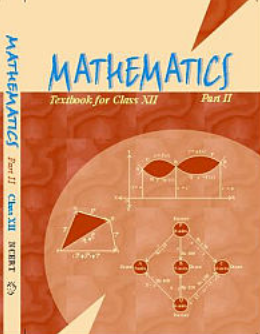•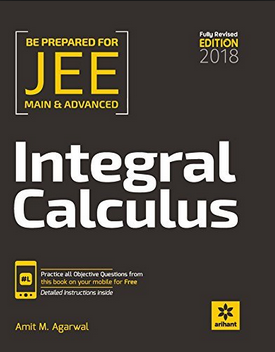Exams
Articles
Questions